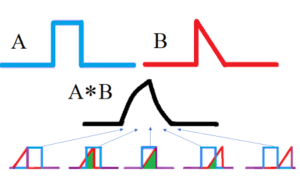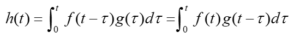# Convolution Integral: Simple Definition

## What is a Convolution Integral?

Mathematically, convolution is an operation on two functions which produces a third combined function;Convolution of two functions A (red) and B (blue) produce a third function describing the overlap (green).

The overlap between the two functions can be evaluated by a convolution integral, which is a “generalized product” of two functions when one of the functions is reversed and shifted.

Other names for the convolution integral include faltung (German for folding), composition product, and superposition integral (Arkshay et al., 2014). These integrals have many applications anywhere solutions for differential equations arise, like engineering, physics, and statistics.

## Formal Definition for Convolution Integral

The convolution integral can be defined as follows (Prasad, 2020):
If the Laplace transforms (F(s) = L{f(t)} and G(s) = L{g(t)}) both exist for s > a ≥ 0, then H(s) = F(s)G(s) = L{h(t)} for s > a, where:The function h(t) is the convolution of f and g. This can also be written simply with a star or asterisk: h(t) = (f * g)(t).

Note that the integral is commutative, i.e. f * g = g * f: it doesn’t matter which function is evaluated first. This useful fact means that you can try placing either of the functions first or second, to see which resulting integral is easier to evaluate.

For example, given the functions x(t) = u(t) and h(t) = u(t)/(t + 1), you could choose one of two forms to evaluate:As convolution is commutative, the integral can be written in two ways. Of the two integrals, (1) is probably the easier to evaluate (U of Washington, 2016).

In general, integrating convolution integrals can be challenging except in the simplest of cases. It can be easier to work first in the Laplace Domain, then transform back into the whatever domain you’re working with, like the time domain (Cheever, 2020).

## References

Behl, A. et al. (2014). Convolution and Applications of Convolution. IJIRT. Volume 1 Issue 6.
Cheever, E. (2020). The Convolution Integral. Retrieved October 18, 2020 from: https://lpsa.swarthmore.edu/Convolution/Convolution.html
Demas, J. (2012). Excited State Lifetime Measurements. Elsevier Science.
Prasad, V. (2020). Ch 6.6: The Convolution Integral. Retrieved October 19, 2020 from: http://faculty.uml.edu/vprasad/oldstuff/92.236.011/ch6.6.ppt
University of Washington (2016). BIOEN 316. Biomedical Signs and Sensors: Solving convolution problems. Retrieved October 19, 2020 from: http://courses.washington.edu/bioen316/Assignments/316_SCP.pdf

CITE THIS AS:
Stephanie Glen. "Convolution Integral: Simple Definition" From StatisticsHowTo.com: Elementary Statistics for the rest of us! https://www.statisticshowto.com/convolution-integral-simple-definition/